# Exploring Numerai Data via R

Numerai is a crowdsourced hedgefund that hosts tournaments, which attract thousands of data scientists around the world to compete for Numeraire cryptocurrency. The company provides clean, regularized, and obfuscated data, where anyone with expertise in machine learning can freely participate. Other than that, they also include various guides and coding samples to help novice data scientists get started. One way to get the data is simply visit https://numer.ai/.

In this article, we will perform exploratory data analysis on the numerai_training_data.csv using R to find out more about each feature and the target. In a recent development, starting for round 238 opening on November 14th, 2020 the official target will migrate from “Kazutsugi” to the newer “Nomi”. In preparation for the migration, we’ll perform our analysis on the new Nomi target.

## The Data

The training_data is 1.3 GB and contains information on 501808 rows across 314 columns. The first three columns are as follow:

• ‘id’ – an unique identifier of each row
• ‘era’ – a time period corresponding to a trading day
• ‘data_type’ – indication of whether the row is part of train/test/validation/live

These three columns are then followed by 310 features columns and the last column for the target. Our very first observation of the data is that all the feature columns and the target column take on only 5 numeric values: 0, 0.25, 0.5, 0.75, and 1. Below shows a sample of the first few columns and rows.

See Code
#Load libraries needed
library(dplyr)
library(mosaic)
library(skimr)
library(ggplot2)
library(GGally)
library(reshape)
library(ppcor)
library(knitr)
library(ggfortify)
library(stringr)
library(gridExtra)
library(tidyr)
library(janitor)

#import data
training_data<-training_and_val_data %>% filter(data_type=="train")
#training_data<-training_data %>% sample_frac(0.01)

id era data_type feature_intelligence1 feature_intelligence2 feature_intelligence3 feature_intelligence4
n000315175b67977 era1 train 0.00 0.5 0.25 0.00
n0014af834a96cdd era1 train 0.00 0.0 0.00 0.25
n001c93979ac41d4 era1 train 0.25 0.5 0.25 0.25

The features are categorized into 6 categories: intelligence, charisma, strength, dexterity, constitution, and wisdom. Note that these categories are not of the same size. There are 12 “Intelligence”, 86 “Charisma”, 38 “Strength”, 14 “Dexterity”, 114 “Constitution”, and 46 “Wisdom” variables. Let’s now take a look the summary statistics of these features.

See Code
#all features
features<-training_data %>%  dplyr::select(starts_with("feature"))

#6 categories of features
intelligence<-training_data %>%  dplyr::select(starts_with("feature_intelligence"))
charisma<-training_data %>%  dplyr::select(starts_with("feature_charisma"))
strength<-training_data %>%  dplyr::select(starts_with("feature_strength"))
dexterity<-training_data %>%  dplyr::select(starts_with("feature_dexterity"))
constitution<-training_data %>%  dplyr::select(starts_with("feature_constitution"))
wisdom<-training_data %>%  dplyr::select(starts_with("feature_wisdom"))

summary<-skim(features)


Table: Data summary

Name features
Number of rows 501808
Number of columns 310
_______________________
Column type frequency:
numeric 5
________________________
Group variables None

Variable type: numeric

skim_variable n_missing complete_rate mean sd p0 p25 p50 p75 p100 hist
feature_intelligence1 0 1 0.5 0.35 0 0.25 0.5 0.75 1 ▇▇▇▇▇
feature_intelligence2 0 1 0.5 0.35 0 0.25 0.5 0.75 1 ▇▇▇▇▇
feature_intelligence3 0 1 0.5 0.35 0 0.25 0.5 0.75 1 ▇▇▇▇▇
feature_intelligence4 0 1 0.5 0.35 0 0.25 0.5 0.75 1 ▇▇▇▇▇
feature_intelligence5 0 1 0.5 0.35 0 0.25 0.5 0.75 1 ▇▇▇▇▇

We see in the data that all the columns are in the correct format, the data is clean and ready for analysis. There are no missing value and from first glance, many of the features share similar distributions. Histograms of the actual values point to an almost universal uniform distribution across each variable, the distributions of the summary statistics appear to be either normally distributed or heavily skewed.

See Code
p1 <- ggplot(summary, aes(x=numeric.mean)) +
geom_histogram()+
labs(title="Distribution of Mean",x="Mean", y = "Frequency")
p2 <- ggplot(summary, aes(x=numeric.sd)) +
geom_histogram()+
labs(title="Distribution of Standard Deviation",x="Standard Deviation", y = "Frequency")
p3 <- ggplot(summary, aes(x=numeric.p25)) +
geom_histogram()+
labs(title="Distribution of First Quartile",x="First Quartile", y = "Frequency")
p4 <- ggplot(summary, aes(x=numeric.p75)) +
geom_histogram()+
labs(title="Distribution of Third Quartile",x="Third Quartile", y = "Frequency")

grid.arrange(p1,p2,p3,p4, ncol=2, nrow=2)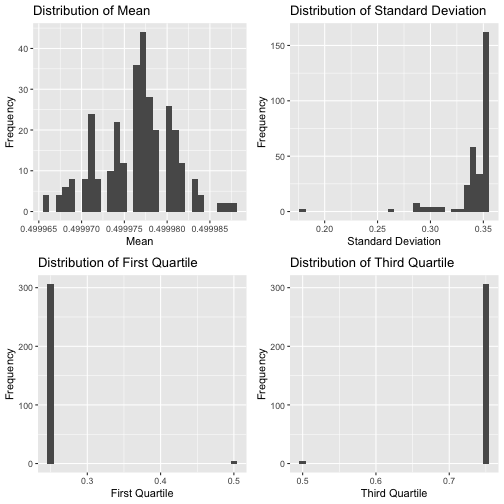It seems that the mean and median of every feature are very close to 0.5. Most of the features have standard deviation around 0.35, while there are a few with exceptionally low standard deviations of less than 0.2. Almost all of the features have their first quartile and third quartile exactly on 0.25 and 0.75 respectfully. A few of them have first quartile and third quartile curiously at 0.50.

How do these distributions look for each feature category? Let’s find out:

See Code
#Create a category column
summary$category<-ifelse(startsWith(summary$skim_variable, 'feature_intelligence') ,'Intelligence',ifelse(startsWith(summary$skim_variable, 'feature_charisma'),'Charisma', ifelse(startsWith(summary$skim_variable, 'feature_strength'), 'Strength', ifelse(startsWith(summary$skim_variable, 'feature_dexterity'), 'Dexterity', ifelse(startsWith(summary$skim_variable, 'feature_constitution'),'Constitution', 'Wisdom')))))

ggplot(summary, aes(x=numeric.mean)) +
geom_histogram()+
labs(title="Distribution of Mean",x="Mean", y = "Frequency") +facet_wrap(~category) +theme(axis.text.x = element_text(angle = 45, vjust = 1,
size = 9, hjust = 1))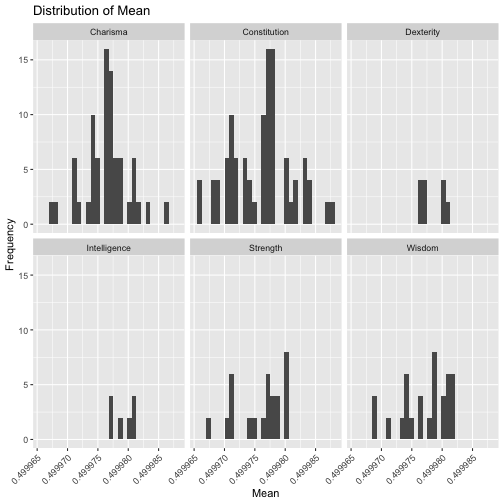See Code
ggplot(summary, aes(x=numeric.sd)) +
geom_histogram()+
labs(title="Distribution of Standard Deviation",x="Standard Deviation", y = "Frequency") +facet_wrap(~category)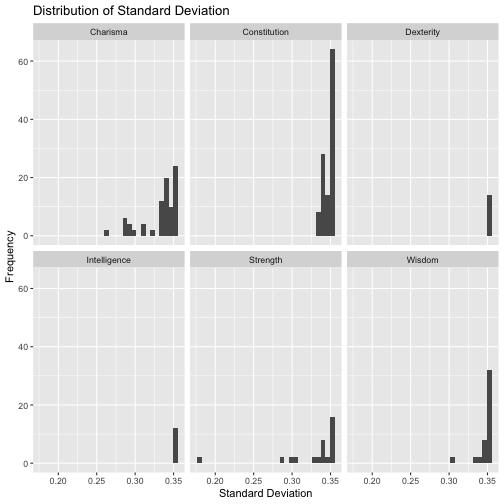We note that outliers seem to appear in the “Strength” and “Charisma” categories. Let’s look more closely to find out which ones.

See Code
#filter to see the outliers
summary %>% filter(numeric.sd<0.2 | numeric.p25==0.5 | numeric.p75==0.5) %>% arrange(numeric.sd)


Table: Data summary

Name features
Number of rows 501808
Number of columns 310
_______________________
Column type frequency:
numeric 4
________________________
Group variables None

Variable type: numeric

skim_variable n_missing complete_rate mean sd p0 p25 p50 p75 p100 hist category
feature_strength20 0 1 0.5 0.18 0 0.5 0.5 0.5 1 ▁▁▇▁▁ Strength
feature_strength38 0 1 0.5 0.18 0 0.5 0.5 0.5 1 ▁▁▇▁▁ Strength
feature_charisma25 0 1 0.5 0.27 0 0.5 0.5 0.5 1 ▂▂▇▂▂ Charisma
feature_charisma47 0 1 0.5 0.27 0 0.5 0.5 0.5 1 ▂▂▇▂▂ Charisma

We can see that feature_strength20 and feature_strength38 are the ones that have the lowest standard deviation (almost half the norm). It is also very interesting that all of the outliers come from two categories: “Charisma” and “Strength”.

Though we have many features, they are all symmetrical and they look like one of the following types.

See Code
h1<-ggplot(features, aes(x=feature_intelligence1))+geom_histogram()+labs(title="Type 1")+ theme(axis.title.x = element_blank())
h2<-ggplot(features, aes(x=feature_constitution2))+geom_histogram()+labs(title="Type 2")+ theme(axis.title.x = element_blank())
h3<-ggplot(features, aes(x=feature_strength29))+geom_histogram()+labs(title="Type 3")+ theme(axis.title.x = element_blank())
h4<-ggplot(features, aes(x=feature_charisma48))+geom_histogram()+labs(title="Type 4")+ theme(axis.title.x = element_blank())
h5<-ggplot(features, aes(x=feature_charisma25))+geom_histogram()+labs(title="Type 5")+ theme(axis.title.x = element_blank())
h6<-ggplot(features, aes(x=feature_strength20))+geom_histogram()+labs(title="Type 6")+ theme(axis.title.x = element_blank())

grid.arrange(h1,h2,h3,h4,h5,h6)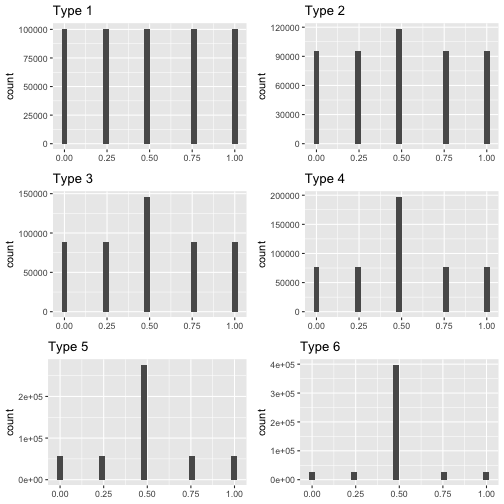Type 1 is uniform distribution and Type 2 to Type 6 are simply various degrees of spread (measured by standard deviation) centered at 0.5. Note that Type 5 and Type 6 are the outliers we mentioned earlier.

We see that the two smallest categories: “Intelligence” and “Dexterity” all have Type 1. “Strength” and “Charisma” have a mix of almost all the types.

Here is a frequency table showing exactly how many of each category is of each Type.

See Code
summary$type<-ifelse(summary$numeric.sd>=0.35, 'Type 1', ifelse(summary$numeric.sd>=0.34 & summary$numeric.sd<0.35, 'Type 2', ifelse(summary$numeric.sd>=0.31 & summary$numeric.sd<0.34, 'Type 3', ifelse(summary$numeric.sd>=0.28 & summary$numeric.sd<0.31, 'Type 4', ifelse(summary$numeric.sd>=0.26 & summary$numeric.sd<0.28, 'Type 5', 'Type 6')))))

category_type<-summary %>% group_by(category) %>% count(type) %>% spread(key = type, value = n) %>% replace_na(list('Type 1' = 0, 'Type 2' =0, 'Type 3' = 0, 'Type 4' =0, 'Type 5' = 0, 'Type 6' =0)) %>% mutate(Total = sum(c_across()))


category Type 1 Type 2 Type 3 Type 4 Type 5 Type 6 Total
Charisma 22 24 24 14 2 0 86
Constitution 62 22 30 0 0 0 114
Dexterity 14 0 0 0 0 0 14
Intelligence 12 0 0 0 0 0 12
Strength 14 6 10 6 0 2 38
Wisdom 30 12 2 2 0 0 46
Total 154 64 66 22 2 2 310

How about the target value? Let’s take a look at it’s summary statistics:

skim(training_data['target'])


Table: Data summary

Name training_data[“target”]
Number of rows 501808
Number of columns 1
_______________________
Column type frequency:
numeric 1
________________________
Group variables None

Variable type: numeric

skim_variable n_missing complete_rate mean sd p0 p25 p50 p75 p100 hist
target 0 1 0.5 0.22 0 0.5 0.5 0.5 1 ▁▃▇▃▁

The target does not belong to any of the aforementioned Types. The distribution looks like a normal distribution.

## Pearson Correlation Coefficient

We now take a look at the correlations between the features.

See Code
cm<-round(cor(features[,1:310]),2) #correlation between features
melted_cm<-melt(cm)
ggplot(data = melted_cm, aes(x=X1, y=X2, fill=value)) +
geom_tile(color = "white")+
scale_fill_gradient2(low = "blue", high = "red", mid = "white",
midpoint = 0, limit = c(-1,1), space = "Lab",
name="Pearson\nCorrelation") +
theme_minimal()+
theme( axis.text.x = element_blank(), axis.text.y= element_blank())Though it is not easy to get much out of the heatmap, we can sort of see that features within each category, they are mostly correlated (some are negative correlated) with each other.

See Code
melted_cm$absvalue<-abs(melted_cm$value)
#sum(melted_cm$value==1) # it's 310 so no feature has correlation 1. melted_cm_filtered<-melted_cm %>% filter(value!=1) %>% arrange(-absvalue) #filtered out correlation 1 and order by absvalue descending order kable(head(melted_cm_filtered,5))  X1 X2 value absvalue feature_wisdom12 feature_wisdom2 0.97 0.97 feature_wisdom2 feature_wisdom12 0.97 0.97 feature_wisdom46 feature_wisdom7 0.96 0.96 feature_wisdom7 feature_wisdom46 0.96 0.96 feature_charisma69 feature_charisma9 0.95 0.95 See Code #filter rows with zero correlation melted_cm_zero<-melted_cm %>% filter(value==0) #look at constitution conzero<-melted_cm_zero %>% filter(grepl('feature_constitution',X1)) %>% filter(grepl('feature_constitution',X2)) #conzero %>% group_by(X2) %>%tally() %>% arrange(-n)  The table confirms that features within the same category are highly correlated with each other (mostly positive correlated). We also see that some features in “Intelligence” are highly correlated with some features in “Dexterity”. For example: • feature_dexterity1 and feature_intelligence10 have correlation 0.82 • feature_dexterity3 and feature_intelligence2 have correlation 0.82 • feature_dexterity8 and feature_intelligence3 have correlation 0.81 On the other hand, we noticed that there are many features that have 0 correlation with each other, and it is surprising that some of them belong to the same category. For example, feature_constitution3 has zero correlation with 14 other features in the same category. We can also explore correlations between linear combinations of features within the same category. Here, we can use equal weighted linear combination (i.e. mean) of the features within each group. Below shows the correlations heatmap: See Code features$intelligence_mean<-rowMeans(intelligence)
features$charisma_mean<-rowMeans(charisma) features$strength_mean<-rowMeans(strength)
features$dexterity_mean<-rowMeans(dexterity) features$constitution_mean<-rowMeans(constitution)
features$wisdom_mean<-rowMeans(wisdom) cm_lc<-round(cor(features[,(ncol(features)-5):ncol(features)]),2) melted_cm_lc<-melt(cm_lc) ggplot(data = melted_cm_lc, aes(x=X1, y=X2, fill=value)) + geom_tile(color = "white")+ scale_fill_gradient2(low = "blue", high = "red", mid = "white", midpoint = 0, limit = c(-1,1), space = "Lab", name="Pearson\nCorrelation") + theme_minimal()+ # minimal theme theme(axis.text.x = element_text(angle = 45, vjust = 1, size = 12, hjust = 1))+ labs(title="Correlations of linear combination of features within a group")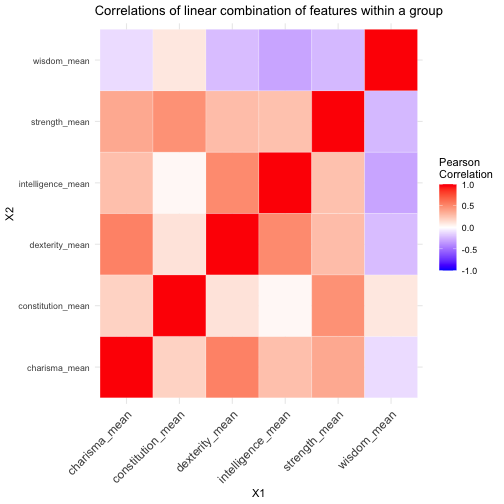We can see that the linear combination of “Intelligence” has no correlation with “Constitution” (0.01). Another interesting observation is that “Dexterity” are moderately correlated with both “Intelligence” and “Charisma” (0.5 and 0.55 respectively) but “Intelligence” and “Charisma” are not very correlated (0.27). We can see the relationships with the scatter plots as well. See Code p5<-ggplot(data = features) + geom_point(mapping = aes(x = intelligence_mean, y = constitution_mean)) p6<-ggplot(data = features) + geom_point(mapping = aes(x = intelligence_mean, y = charisma_mean)) p7<-ggplot(data = features) + geom_point(mapping = aes(x = intelligence_mean, y = dexterity_mean)) p8<-ggplot(data = features) + geom_point(mapping = aes(x = charisma_mean, y = dexterity_mean)) grid.arrange(p5,p6,p7,p8, ncol=2, nrow=2)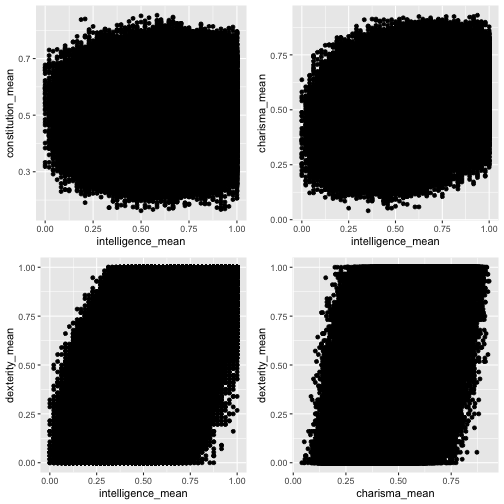When we look at the correlations between the features and the target, we see that all of them are not correlated with the target. The highest correlation of 0.01/-0.01 with the target. This simply implies that the features do not have a linear relationship with the target. What it does not imply is that the features have no relationship with the target. See Code cm_target<-round(cor(training_data[,4:314]),2) melted_cm_target<-melt(cm_target) %>% filter(X2=='target') melted_cm_target$absvalue<-abs(melted_cm_target$value) kable(head(melted_cm_target %>% arrange(-absvalue),5))  X1 X2 value absvalue target target 1.00 1.00 feature_intelligence2 target -0.01 0.01 feature_intelligence3 target -0.01 0.01 feature_charisma1 target 0.01 0.01 feature_charisma2 target 0.01 0.01 As expected, when we look at correlations between linear combinations of features and the target, we see that none of them are correlated with the target. See Code cm_target_lc<-round(cor(features[,(ncol(features)-5):ncol(features)],training_data$target),2)
kable(cm_target_lc)

intelligence_mean 0.00
charisma_mean 0.01
strength_mean 0.01
dexterity_mean -0.01
constitution_mean 0.00
wisdom_mean 0.01

## Scatter Plots

Looking at many of the scatter plots, almost all of the scatter plots look like the following, even the ones with high correlation. Unfortunately, we are not able to say much about the features from the scatter plots other than they have taken almost all possible combinations of values in the data.

pairs(intelligence[1:5])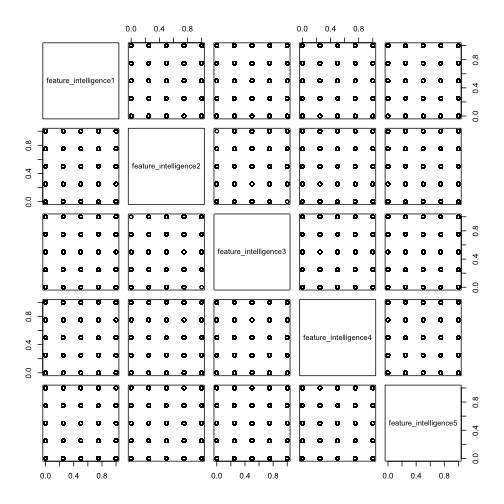## Mosaic Plots

Let’s explore some mosaic plots. For instance, going back to the outlier we discussed, this mosaic plot shows that feature_strength20 are heavily centered at 0.5 while feature_intelligence1 is evenly distributed at each value.

mosaicplot(~feature_strength20+feature_intelligence1,data=features)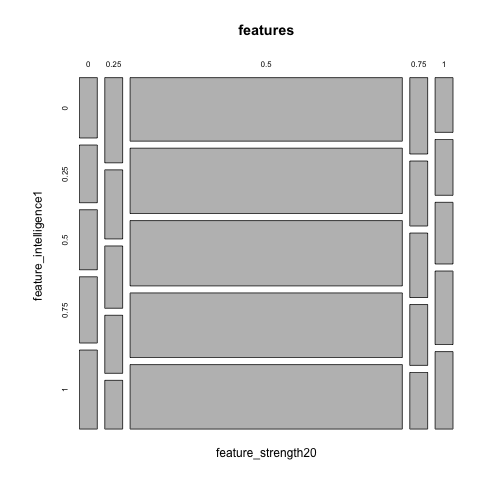Here is another interesting mosaic plot where it clearly shows that there is a strong correlation between feature_intelligence10 and feature_dexterity1, which we have seen while we looked at correlations.

mosaicplot(~feature_intelligence10+feature_dexterity1,data=features)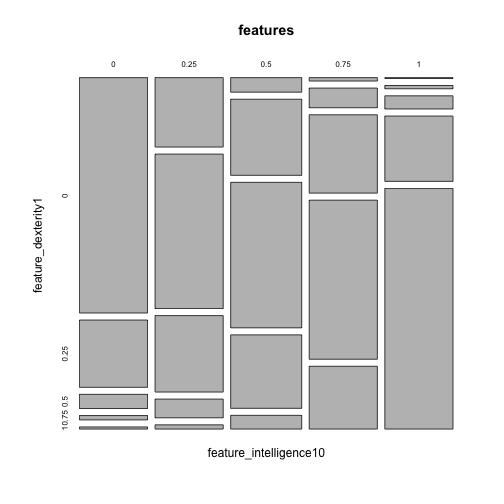## Principal Components Analysis

Since we have many features, principal component analysis could be useful. We see that the first 50 principal components will be able to explain 82% of variance, which is not bad considering we started from 310 features.

See Code
pca<-prcomp(features[,1:310], scale=TRUE) #pca
VE<-pca$sdev^2 pca_tve<-round(VE/sum(VE),2) pca_tve[1:10]  ##  0.10 0.08 0.05 0.04 0.03 0.03 0.02 0.02 0.02 0.02  Here are two plots of PC1 vs PC2, where no clear clusters can be seen. Notice that similar situation occurs across the rest of the components as well. We can only conclude that the target value exists all across different inputs, which is unfortunate as it implies predicting the target could be a difficult task. See Code training_data$target_factor=factor(training_data$target) x <- pca$x %>%
as_tibble() %>%
mutate(target=training_data\$target_factor)

p9<-ggplot(data = x, aes(x = PC2, y = PC1, colour =target)) +
geom_point()

p10<-ggplot(data = x, aes(x = PC2, y = PC1, colour =target)) +
geom_point()+facet_wrap(~target)

p9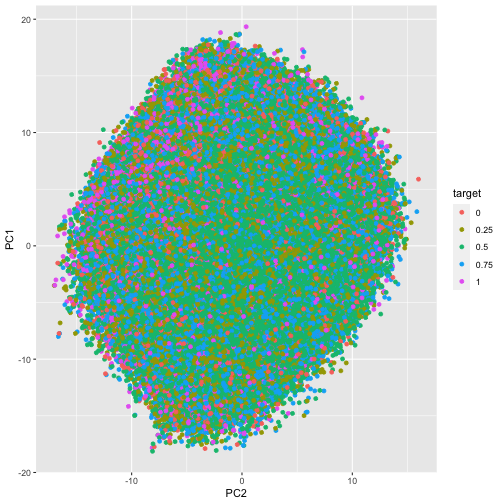See Code

p10